# HBSE 8th Class Maths Solutions Chapter 16 Playing with Numbers Ex 16.1

Haryana State Board HBSE 8th Class Maths Solutions Chapter 16 Playing with Numbers Ex 16.1 Textbook Exercise Questions and Answers.

## Haryana Board 8th Class Maths Solutions Chapter 16 Playing with Numbers Exercise 16.1

Question 1.
Find the values of the letters in each of the following and give reasons for the steps involved.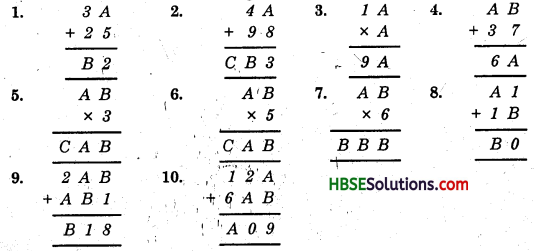Solution:
1. We have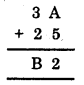Study the addition in the ones column: from A + 5, we get ‘2’ that is, a number whose ones digit is 2.
Thus, A should be 7. So the puzzle can be solved as shown below :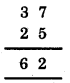That is, B = 6.

2. We have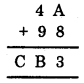Here, A + 8 = 3 (a number whose ones digit is 3)
∴ ‘A’ should be 5.
Now,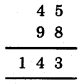Thus, A = 5, B = 4 and C = 1.

3. We have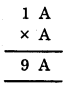Since the ones digit of, A × A = A, so, it must be 6, because since produce of a number and A is 9.
i.e. 1 × A = 9 ⇒ A = 9
but A = 9 does not satisfies.
So, A = 6
Now,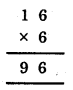4. We have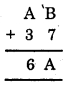Here, B + 7 is A’ that is a number whose ones digit is ‘A’.
Let us put the values of ‘B’ starting from 0.
If B = 0, then A = 7 ∴ 7 + 6 ≠ 6
If B = 1, then A = 8 ∴ 8 + 3 ≠ 6
If B = 2, then A = 9 ∴ 9 + 3 ≠ 6
If B = 5, then A = 2 ∴ (2 + 3) + 1 = 6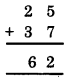So, B = 5 and A = 2 satisfies the given condition.

5. We have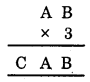Since the ones digit of B × 3 is B, it must be B = 0 or B = 5.
Now look at A.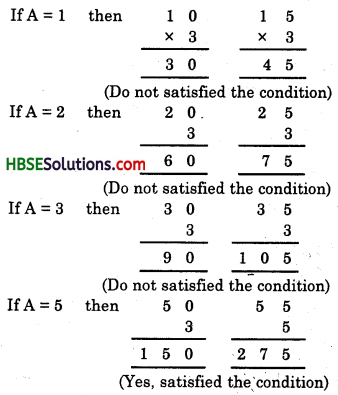So, B = 0 and A = 5 and C = 1.

6. We have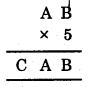Since the ones digit of B × 5 is B
∴ B must be 0 or 5
Now, look at A.
Use hits and trial method :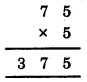∴ A = 7, B = 5 and C = 3.7. We have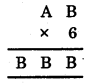Since the ones digit of B × 6 is 6.
∴ Possible values of B are 0, 4, 6 and 8.
Now, look at A.
So, the value of BBB may be 444,666 or 888. [000 is not possible]
∴ 666 ÷ 6 = 111
which is a 3-digits number.
888 ÷ 6 = 148
which is a 3-digits number.
444 ÷ 6 = 74
which is a 2-digits number.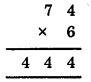Thus, B = 4 and A = 7.

8. We have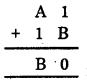If you study the addition in the ones column i.e. (1 + B), you get ‘0’.
∴ B should be 9.
Thus, A must be 7.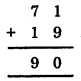Thus, B = 9 and A = 7.

9. We have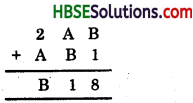If you study the addition in the ones column i.e. (B + 1) then you get ‘8’.
∴ ‘B’ should be 7 and A should be 4.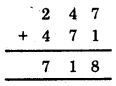So, the given condition is satisfied.
Hence, B = 7 and A = 4.10. We have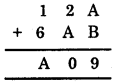Since addition of ones column gives the value 9.
∴ A + B should be (1, 8) or (8, 1).
Now, let us apply hits and trial method.
If A = 1 and B = 8, then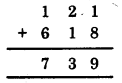If A = 8 and B = 1, then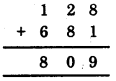Thus, A = 8 and B = 1 satisfies the given condition.